Get instant live expert help with Excel or Google Sheets“My Excelchat expert helped me in less than 20 minutes, saving me what would have been 5 hours of work!”

#### Post your problem and you’ll get Expert help in seconds.

Your message must be at least 40 characters
Our professional Expert are available now. Your privacy is guaranteed.

# Get months between dates

To measure a timeline for an activity we calculate the difference between two dates in terms of days, weeks, months and years. But how can we calculate the difference between two dates in months in Excel? There are different formulas to get months between two dates in Excel.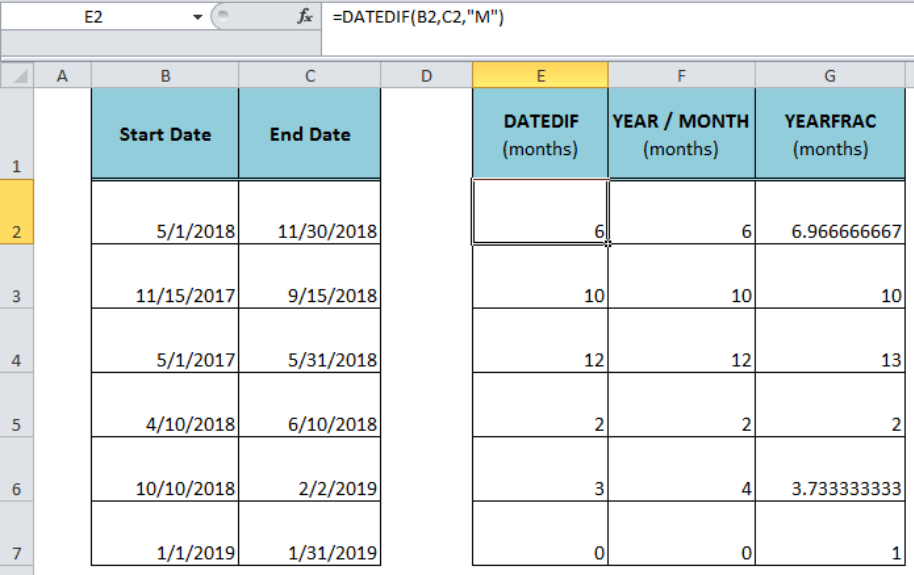Figure 1. Calculating Months Between Two Dates

## Using the Excel DATEDIF Formula

The Excel DATEDIF function calculates the difference between two dates based on the interval specified like days, months, years. Using this function we can return the number of complete months between two dates as per the following syntax;

`=DATEDIF( start_date, end_date, “M”)`

The DATEDIF function rounds down the values by default and returns the complete number of months between dates. In our example, we can get months between two dates listed in column B and C as start date and end date values. We need to use the following formula in cell E2 and drag the fill handle down;

`=DATEDIF(B2,C2,"M")`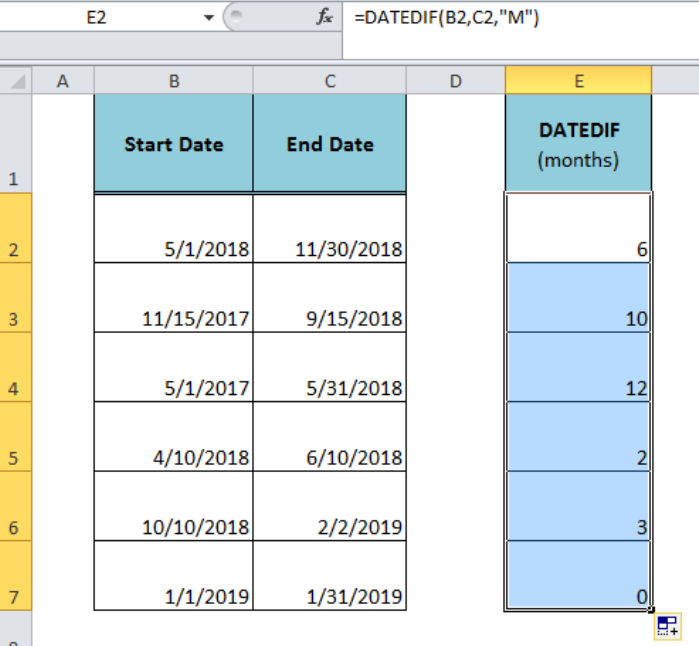Figure 2. Using the DATEDIF Function to Calculate Months Between Two Dates

## Using the YEAR and MONTH Formula

We can calculate months between two dates in Excel using the YEAR and MONTH functions in a formula as per the following syntax;

`=(YEAR(end_date)-YEAR(start_date))*12+MONTH(end_date)-MONTH(start_date)  `

This formula compares two given dates and calculates months between two dates either as a positive or negative number. We will apply this formula in cell F2 and copy the formula down, such as;

`=(YEAR(C2)-YEAR(B2))*12+MONTH(C2)-MONTH(B2)`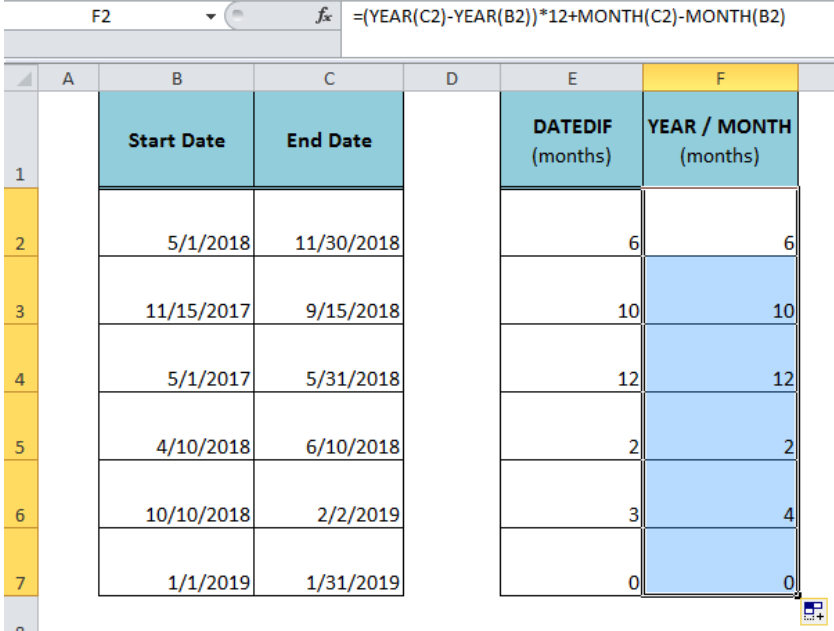Figure 3. Using the YEAR and MONTH Formula to Calculate Months Between Dates

Notice that the result of this formula is not always similar to the DATEDIF function. Because, the DATEDIF function returns the number of complete months elapsed between dates, and this formula works on the months’ numbers that belong to different dates. Like in row 6, the DATEDIF function returns 3 months and the YEAR/MONTH formula returns 4 because the dates belong to two different months.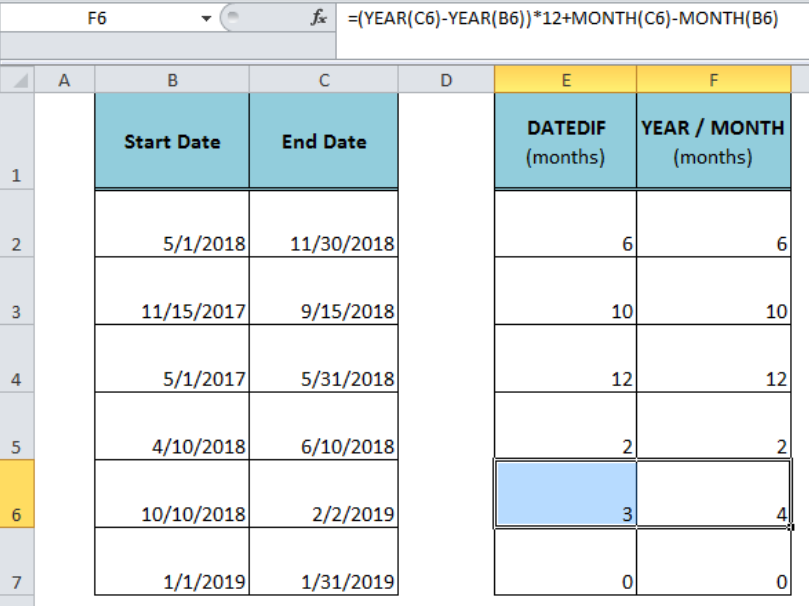Figure 4. The Difference Between DATEDIF and YEAR/MONTH Formulas

## Using the YEARFRAC Formula

The Excel YEARFRAC function returns the year fraction representing the number of whole days between two dates. Using this function we can return the number of fractional months or absolute months between two dates as per the following syntax;

`=YEARFRAC( start_date, end_date) * 12`

In this example, we will use the following formula in cell G2 to get fractional months between two dates and copy it down to below cells;

`=YEARFRAC(B2,C2)*12`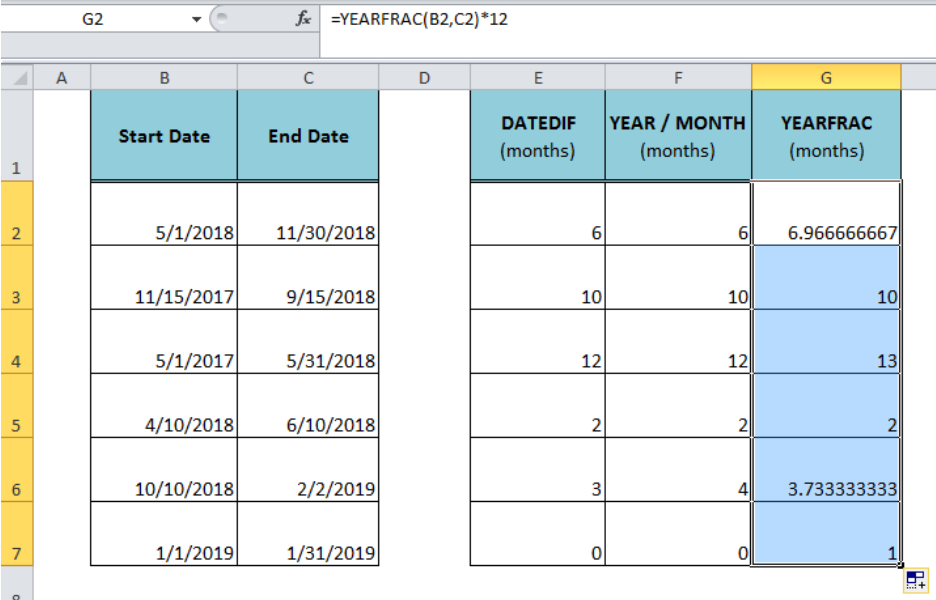Figure 5. Using the YEARFRAC Formula to Calculate Months Between Dates

## Instant Connection to an Expert through our Excelchat Service:

Most of the time, the problem you will need to solve will be more complex than a simple application of a formula or function. If you want to save hours of research and frustration, try our live Excelchat service! Our Excel Experts are available 24/7 to answer any Excel question you may have. We guarantee a connection within 30 seconds and a customized solution within 20 minutes.

### Did this post not answer your question? Get a solution from connecting with the expert.Another blog reader asked this question today on Excelchat:
Solution exampleshow do I derive the monthly sales from a date and revenue column
Solved by A. U. in 23 minsI need a datedif function that calculates the difference in 2 dates and if it returns more than 5 years, I need it to say yes and if not no.
Solved by O. Y. in 14 minsa date formula that results in showing the date as 29/12/00 (date format as dd/mm/yy) - use column BA to calculate 364 days (same date format) in column BS, if BA blank, then calculate 364 days using column AX. Results for column BA are correct. However, if column BA is empty, then it should calculate using column AX, which has data, but the result is always 29/12/00, regardless of the date in column AX. I have used this formula with success in another workbook, but this file wont work! Formula: =IF(AND(LEN(\$BA74)=0,LEN(\$AX74)=0),"",IF(LEN(\$BA74)=0,DATE(YEAR(\$AX74),MONTH(\$AX74),DAY(\$AX74)+364),DATE(YEAR(\$BA74),MONTH(\$BA74),DAY(\$BA74)+364)))
Solved by A. L. in 60 minsHi there. I need a column to automatically calculate the numbers of days from a start time to today's date.
Solved by T. F. in 16 minsI need a formula that looks up if the date (which looks like "8/1/2018 8:05:36 AM") in Column "C" contains year 2018. And then populates "2018" in Column D, if the date does not contain "2018" then enter False in Column D.
Solved by F. H. in 20 mins## Subscribe to Excelchat.coAnother blog reader asked this question today on Excelchat: